# De la réussite au brevet dans cinq collèges rennais

Ce matin, on a travaillé sur des données de réussite au brevet des collèges, sur Rennes (dans le cadre du cours de statistique). On dispose de données pour 5 collèges rennais, sur une douzaine d’années (entre 2001 et 2015).

• Analyse de le temps de l’agrégation des collèges

On avait commencé par quelque chose de simple: regarder l’évolution dans le temps du taux de réussite,

> base=
> idx=seq(4,ncol(base),by=3)
> annees=as.numeric(substr(names(base)[idx],2,5))
> College=6
> plot(annees,base[College,idx],type="b")# Comparer des taux sur deux populations

Lors du dernier cours, nous avions évoqué les tests de comparaison des moyennes et des proportions sur deux échantillons. Pour cela, nous avions vu qu’il était possible d’utiliser la statistiqueLa statistique doit alors suivre, sous l’hypothèse où les proportions sont égales une loi normale centrée réduite. Sous R, c’est assez facile à implémenter. Afin d’illustrer, utiliser l’information suivanteBon, en l’état on n’a pas vraiment un taux, mais un nombre de tués sur les routes. On peut introduire la probabilité d’avoir un tué sur les routes par minute. Le code sous R serait alors

> prop.test(x=c(308,308/1.027), n=c(31*24*60,31*24*60), alt="two.sided")

2-sample test for equality of proportions with continuity correction

data:  c(308, 308/1.027) out of c(31 * 24 * 60, 31 * 24 * 60)
X-squared = 0.0834, df = 1, p-value = 0.7727
alternative hypothesis: two.sided
95 percent confidence interval:
-0.0009198487  0.0012826342
sample estimates:
prop 1      prop 2
0.006899642 0.006718249

Autrement dit, la probabilité d’avoir un accident, à la minute, est sensiblement le même, avec une p-value de 77%. On notera sur le graphique suivant que l’impact du pas de temps a globalement peu d’importance….)L’idéal serait d’avoir des données détaillées, mais il faudra attendre un peu pour ça…

# STT2700, estimation, tests et coupes du mondeMercredi, pour le dernier cours, nous allons revenir sur l’estimation, les tests, et plus généralement sur la modélisation statistique. Pour cela, j’avais pensé travailler sur les nombres de buts marqués, par match, lors de différentes coupes du monde de soccer (1982, 1998 et 2010). Je ne mets pas l’intégralité du code aujourd’hui, l’idée est pour l’instant de mettre en ligne des données qui serviront à répondre aux questions qui seront posées mercredi. Le code (accompagné – éventuellement – d’explications théoriques) sera posté par la suite.

soccer1982=read.table("http://freakonometrics.free.fr/soccer1982")
S82=(soccer1982$V1+soccer1982$V2)
S98=(soccer1998$V1+soccer1998$V2)
S10=(soccer2010$V1+soccer2010$V3)

Les boxplot associés à ces trois échantillons sont les suivants,On va se poser des questions autour de ces données, par exemple voir s’il est vraisemblance (ou pas) que le nombre moyen de but dans un match (avant prolongation, s’il y en a eu). On peut commencé par essayer de se demander quel modèle utiliser. Classiquement, la loi de Poisson est la plus utilisée (en plus, c’est la seule loi qui est autorisée lorsqu’on publie un billet le 1er avril). Les histogrammes sont les suivants

boxplot(S82,S98,S10,col=c("red","yellow","blue"),
label=("1982","1998","2010"))
hist(S82,breaks=0:11,col="red")
hist(S98,breaks=0:11,col="yellow")
hist(S10,breaks=0:11,col="blue")Si on compare les fonctions de répartition empiriques à celles de lois de Poisson ajustées par maximum de vraisemblance, on obtient, pour la coupe du monde de 1982et pour celle de 2010,Visuellement, l’ajustement semble relativement bon, surtout en 2010. On peut aussi faire un test du chi-deux,

> library(vcd)
> (GF=goodfit(S10,type="poisson"))

Observed and fitted values for poisson distribution
with parameters estimated by ML

count observed     fitted
0        7  6.6409703
1       17 15.0459484
2       13 17.0442384
3       14 12.8719508
4        7  7.2907534
5        5  3.3036226
6        0  1.2474617
7        1  0.4037543

> summary(GF)

Goodness-of-fit test for poisson distribution

X^2 df  P(> X^2)
Likelihood Ratio 5.586765  5 0.3485255

On voit que l’on accepte l’ajustement par une loi de Poisson. Pour ceux qui veulent une visualisation, sur la figure ci-dessous, on a la densité d’une loi du chi-deux. Le premier trait vertical est la valeur observée, et l’aire jaune est alors la p-value (qui excède largement 5%). En rouge on a 5%, donc le second trait vertical est la borne de la région critique associé au test pour une erreur de première espèce valant 5%,On peut ensuite faire plein de tests.  On suppose que . On va pouvoir tester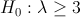contre une hypothèse alternative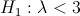Comme on a une hypothèse sur la loi des observations qui semble robuste, on peut utiliser un test de type rapport de vraisemblance.
On peut aussi faire un test de la forme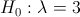contre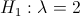(histoire de tester des hypothèses simples – qui ont une interprétation). Sinon, comme ce qui nous intéresse, c’est de savoir si on a plus de trois buts par matchs, on peut définir la variable binomiale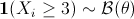, en notant que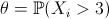est une proportion – donc facile à tester – qui nous intéresse ici compte tenu du problème que l’on cherchera à résoudre. On pourra alors tester, par exemple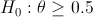contre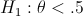Ces derniers tests sont alors facile à mettre en œuvre,

> Z=(S10>=3)*1
> prop.test(sum(Z),length(Z),p=1/2,alternative="less")

1-sample proportions test with continuity correction

data:  sum(Z) out of length(Z), null probability 1/2
X-squared = 1.2656, df = 1, p-value = 0.1303
alternative hypothesis: true p is less than 0.5
95 percent confidence interval:
0.0000000 0.5322764
sample estimates:
p
0.421875

On peut aussi faire des tests de moyenne sur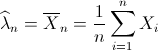Un test de l’hypothèsecontre une hypothèse alternatives’écrit alors
> t.test(S10,mu=3,alternative ="less")

One Sample t-test

data:  S10
t = -3.7763, df = 63, p-value = 0.0001775
alternative hypothesis: true mean is less than 3
95 percent confidence interval:
-Inf 2.590273
sample estimates:
mean of x
2.265625
Mais on l’aura l’occasion de revoir tous les points du cours, y compris aller peut être un peu plus loin, par exemple sur la comparaison de moyenne entre échantillons,
> t.test(S82,S98,var.equal=FALSE)

Welch Two Sample t-test

data:  S82 and S98
t = 0.427, df = 85.266, p-value = 0.6704
alternative hypothesis: true difference in means is not equal to 0
95 percent confidence interval:
-0.5503669  0.8514658
sample estimates:
mean of x mean of y
2.807692  2.657143

# Want to say one thing and the exact oppositive with strong confidence ?

No need to do politics. Just take a statistical course. And I do not talk about misinterpretation of statistics, but I talk about the mathematical foundations of statistical tests.
Consider the following parametric test, with a one-dimensional parameter:versus, for some (fixed). A standard way of doing such a test is to consider an rejection region. The test works as follows: consider a sample,

• if, then we accept• if, the we rejectFor instance, consider the case of a Bernoulli sample, with probability. The standard idea is to defineThe rejection region is then based on statistic,

• if, then we accept• if, the we rejectwhere thresholdis taken so that the probability to make a first type error is(say 5%) using the Gaussian approximation for z. HereThus, the acceptation region is then the green area below, while the rejection region is the red one, for.Consider now the exact opposite test (with the same),versus. Here, we use the same statistics, and the test is

• if, then we accept• if, the we rejectwhere nowThus, now, the acceptation region is then the green area below, while the rejection region is the red one.So if we summarize what we just said,

• in the region on the left below, both test agree that• in the region on the right below, both test agree that• and in the region in blue, in the middle, the two tests disagree (one claims that, and the other one that)Here is the evolution of the region as a function of(the size of the sample) when the sample frequency is 20%. With a small sample size, we can hardly say anything.

n=seq(1,100)
p=0.2
x1=p+qnorm(.95)*sqrt(p*(1-p)/n)
x2=p+qnorm(.05)*sqrt(p*(1-p)/n)
plot(n,x1,type="l",ylim=c(0,1))
polygon(c(n,rev(n)),c(x1,rev(x2)),col="light blue",border=NA)
lines(n,x1,lwd=2,col="red")
lines(n,x2,lwd=2,col="red")One might say that those bounds are based on a Gaussian approximation which is not correct whenis too small. So we can compute exact bounds,
y1=qbinom(.95,size=n,prob=p)/n
y2=qbinom(.05,size=n,prob=p)/n
polygon(c(n,rev(n)),c(y1,rev(y2)),col="blue",border=NA)
lines(n,y1,lwd=2,col="red")
lines(n,y2,lwd=2,col="red")

and we getThis is what we can observe if we use R statistical procedures, either the asymptotic one,

> prop.test(2,10,.5,alternative="less")

1-sample proportions test with continuity correction

data:  2 out of 10, null probability 0.5
X-squared = 2.5, df = 1, p-value = 0.05692
alternative hypothesis: true p is less than 0.5
95 percent confidence interval:
0.0000000 0.5100219
sample estimates:
p
0.2

> prop.test(2,10,.5,alternative="greater")

1-sample proportions test with continuity correction

data:  2 out of 10, null probability 0.5
X-squared = 2.5, df = 1, p-value = 0.943
alternative hypothesis: true p is greater than 0.5
95 percent confidence interval:
0.04368507 1.00000000
sample estimates:
p
0.2

or a more accurate one

> binom.test(2,10,.5,alternative="less")

Exact binomial test

data:  2 and 10
number of successes = 2, number of trials = 10, p-value = 0.05469
alternative hypothesis: true probability of success is less than 0.5
95 percent confidence interval:
0.0000000 0.5069013
sample estimates:
probability of success
0.2

> binom.test(2,10,.5,alternative="greater")

Exact binomial test

data:  2 and 10
number of successes = 2, number of trials = 10, p-value = 0.9893
alternative hypothesis: true probability of success is greater than 0.5
95 percent confidence interval:
0.03677144 1.00000000
sample estimates:
probability of success
0.2Here, when the sample frequency is 20% andis equal to 10, we accept at the same time that theta is higher than 50% and lower than 50%.
And obviously it is not only a theoretical problem: it has obviously some strong implications. This morning, a good friend mentioned a post published some months ago, online here, about discrimination, and the lack of women with academic positions in mathematics, in France. As claimed by the author of the post“A Paris VI, meilleure université française selon son président, sur 11 postes de maitres de conférences, 5 filles classées premières. Il y a donc des filles excellentes ? A Toulouse, sur 4 postes, 2 filles premières. Parité parfaite. Mais à côté de cela, Bordeaux, 4 postes, 0 fille première. Littoral, 3 postes, 0 fille, Nice, 5 postes, 0 fille, Rennes, 7 postes, 0 fille…”.
Consider the latter one: in Rennes, out of 7 people hired last year, no woman. So in some sense, it looks obvious that there is some kind of discrimination ! Zero out of seven ! Well, if we consider the fact that around 30% of PhD thesis in mathematics were defended by women those years, we can also try to see is there if no “positive discrimination“, i.e. testwhere theta is the probability to hire a woman (just to be a little bit provocative).

> prop.test(0,7,.3,alternative="less")

1-sample proportions test with continuity correction

data:  0 out of 7, null probability 0.3
X-squared = 1.7415, df = 1, p-value = 0.09347
alternative hypothesis: true p is less than 0.3
95 percent confidence interval:
0.0000000 0.3719021
sample estimates:
p
0

Warning message:
In prop.test(0, 7, 0.3, alternative = "less") :
Chi-squared approximation may be incorrect
> binom.test(0,7,.3,alternative="less")

Exact binomial test

data:  0 and 7
number of successes = 0, number of trials = 7, p-value = 0.08235
alternative hypothesis: true probability of success is less than 0.3
95 percent confidence interval:
0.0000000 0.3481637
sample estimates:
probability of success
0

With no woman hired that year, we can still pretend that there was some kind of “positive discrimination“. An note that we do accept – with more confidence – the assumption of “positive discrimination” if we look at all universities together,

> prop.test(5+2,11+4+4+3+5+7,.3,alternative="less")

1-sample proportions test with continuity correction

data:  5 + 2 out of 11 + 4 + 4 + 3 + 5 + 7, null probability 0.3
X-squared = 1.021, df = 1, p-value = 0.1561
alternative hypothesis: true p is less than 0.3
95 percent confidence interval:
0.0000000 0.3556254
sample estimates:
p
0.2058824

> binom.test(5+2,11+4+4+3+5+7,.3,alternative="less")

Exact binomial test

data:  5 + 2 and 11 + 4 + 4 + 3 + 5 + 7
number of successes = 7, number of trials = 34, p-value = 0.1558
alternative hypothesis: true probability of success is less than 0.3
95 percent confidence interval:
0.0000000 0.3521612
sample estimates:
probability of success
0.2058824

So obviously, with small sample, almost anything can be claimed !